# Zariski tangent space

(diff) ← Older revision | Latest revision (diff) | Newer revision → (diff)

to an algebraic variety or schemeat a pointThe vector space over the residue field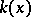of the pointthat is dual to the space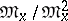, where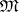is the maximal ideal of the local ringofon. Ifis defined by a system of equationswhere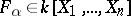, then the Zariski tangent space at a rational pointis defined by the system of linear equations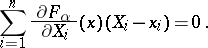A varietyis non-singular at a rational pointif and only if the dimension of the Zariski tangent space toatis equal to the dimension of. For a rational point, the Zariski tangent space is dual to the space— the stalk atof the cotangent sheaf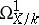. An irreducible varietyover a perfect fieldis smooth if and only if the sheafis locally free. The vector bundle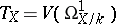associated withis called the tangent bundle ofover; it is functorially related to. Its sheaf of sections is called the tangent sheaf to. The Zariski tangent space was introduced by O. Zariski .

How to Cite This Entry:
Zariski tangent space. Encyclopedia of Mathematics. URL: http://encyclopediaofmath.org/index.php?title=Zariski_tangent_space&oldid=13053
This article was adapted from an original article by V.I. Danilov (originator), which appeared in Encyclopedia of Mathematics - ISBN 1402006098. See original article